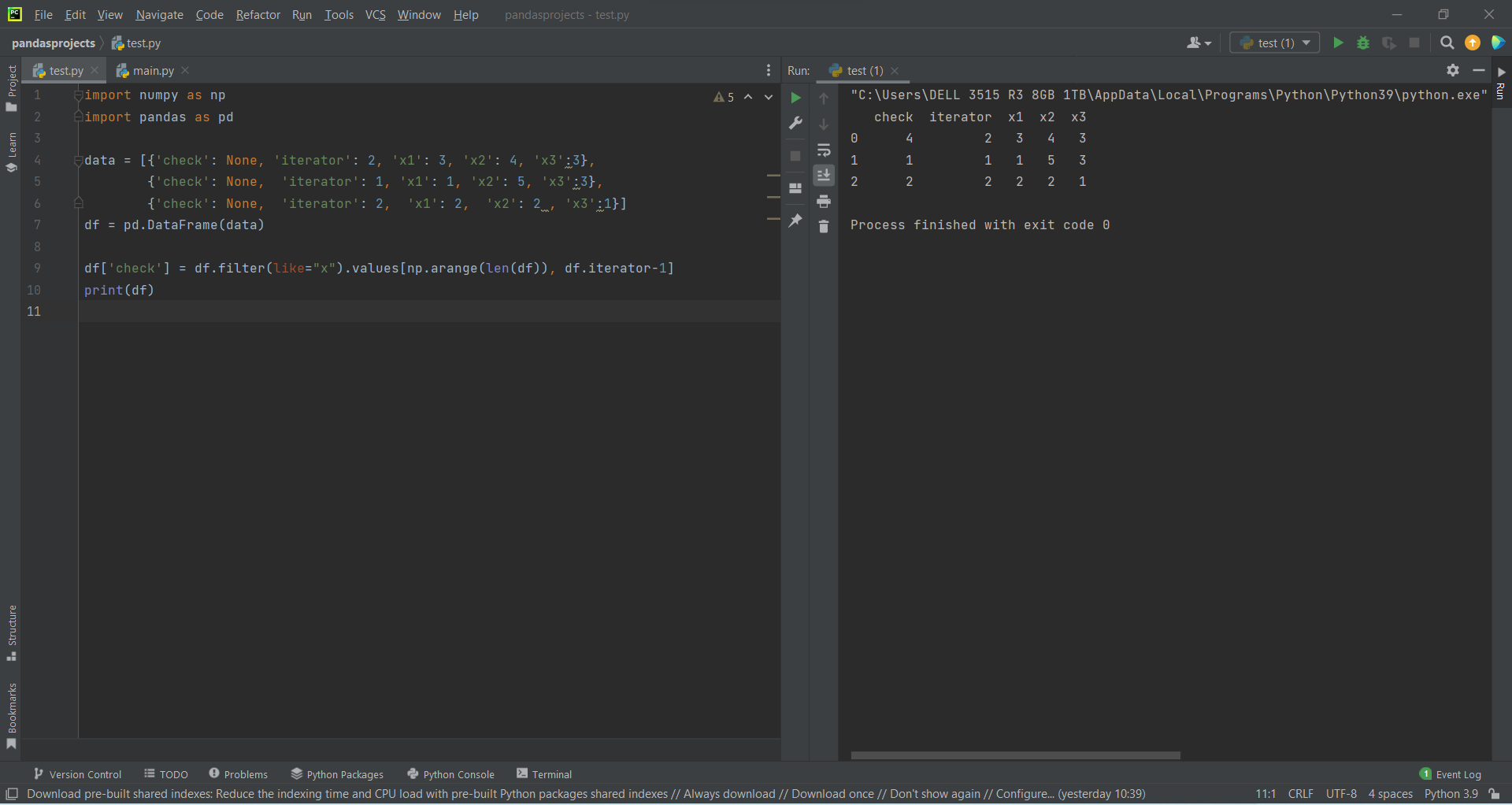# Select Pandas Dataframe Column Value based on Different Column Valueby Abdul Rawoof A RUpdated: Feb 10, 2023Solution Kit

In pandas, a DataFrame column refers to a single data series within a DataFrame. Each column has a unique name and contains a specific set of data. The columns of a DataFrame can be accessed using the column name as an attribute of the DataFrame, or by using the column name as a key in a dictionary-like notation.

You can also perform various operations on a DataFrame column, such as mathematical, logical, string, etc. For example, you can add, subtract, multiply, divide, or apply any mathematical function to the entire column.

In pandas, you can select specific rows of a DataFrame based on the value of a different column.

• You can use the “.loc[]” attribute.
• Using the & (and) or | (or) operators, you can also chain multiple conditions together.
• You can also select specific columns along with the condition.
• You can also use other comparison operators like >, <, >=, <=, != ,etc.

To know more about selecting Pandas DataFrame column values based on different values, refer to the code below.Fig : Preview of the output that you will get on running this code from your IDE.

### Code

In this solution we're using Pandas and NumPy libraries.

### Instructions

Follow the steps carefully to get the output easily.

2. Copy the snippet using the 'copy' and paste it in your IDE.
3. Add required dependencies and import them in Python file(import pandas & numpy).
4. Remove the first line in the code after pasting it in your IDE(refer preview of the output).
5. Add print statement at end of the code('print(df)' instead of 'df').
6. Run the file to generate the output.

I hope you found this useful. I have added the link to dependent libraries, version information in the following sections.

I found this code snippet by searching for 'Select Pandas Dataframe Column Value Based on Different Column Value' in kandi. You can try any such use case!

### Environment Tested

I tested this solution in the following versions. Be mindful of changes when working with other versions.

1. The solution is created in PyCharm 2021.3.
2. The solution is tested on Python 3.9.7.
3. Pandas version-v1.5.2.
4. NumPy version-v1.24.1.

Using this solution, we are able to select dataframe column value based on different column value with simple steps. This process also facilities an easy way to use, hassle-free method to create a hands-on working version of code which would help us to select dataframe column value based on different column value.

### Dependent Libraries

pandasby pandas-dev

Python38552Version:v2.0.2Flexible and powerful data analysis / manipulation library for Python, providing labeled data structures similar to R data.frame objects, statistical functions, and much more

Support
Quality
Security
Reuse

pandasby pandas-dev

Python38552Version:v2.0.2License: Permissive (BSD-3-Clause)

Flexible and powerful data analysis / manipulation library for Python, providing labeled data structures similar to R data.frame objects, statistical functions, and much more
Support
Quality
Security
Reuse

numpyby numpy

Python23663Version:v1.25.0rc1The fundamental package for scientific computing with Python.

Support
Quality
Security
Reuse

numpyby numpy

Python23663Version:v1.25.0rc1License: Permissive (BSD-3-Clause)

The fundamental package for scientific computing with Python.
Support
Quality
Security